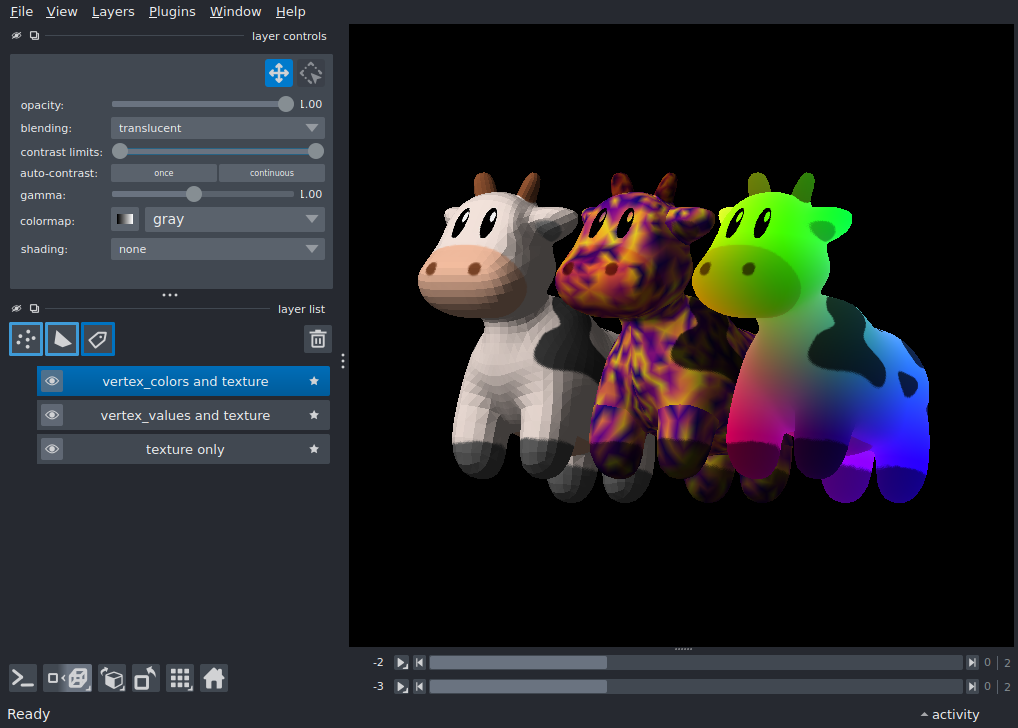# Surface with texture and vertex_colors¶

Display a 3D surface with both texture and color maps.

This example demonstrates how surfaces may be colored by:
• setting vertex_values, which colors the surface with the selected colormap

• setting vertex_colors, which replaces/overrides any color from vertex_values

• setting both texture and texcoords, which blends a the value from a texture (image) with the underlying color from vertex_values or vertex_colors. Blending is achieved by multiplying the texture color by the underlying color - an underlying value of “white” will result in the unaltered texture color.

Tags: visualization-nD```import numpy as np

import napari

# load the model and texture
vertices, faces, _normals, texcoords = read_mesh(mesh_path)
n = len(vertices)

flat_spot = napari.layers.Surface(
(vertices, faces),
translate=(1, 0, 0),
texture=texture,
texcoords=texcoords,
name="texture only",
)

np.random.seed(0)
plasma_spot = napari.layers.Surface(
(vertices, faces, np.random.random((3, 3, n))),
texture=texture,
texcoords=texcoords,
colormap="plasma",
name="vertex_values and texture",
)

rainbow_spot = napari.layers.Surface(
(vertices, faces),
translate=(-1, 0, 0),
texture=texture,
texcoords=texcoords,
# the vertices are _roughly_ in [-1, 1] for this model and RGB values just
# get clipped to [0, 1], adding 0.5 brightens it up a little :)
vertex_colors=vertices + 0.5,
name="vertex_colors and texture",
)

# create the viewer and window
viewer = napari.Viewer(ndisplay=3)